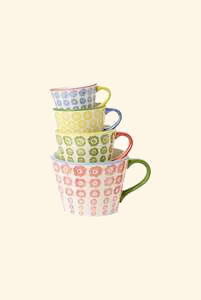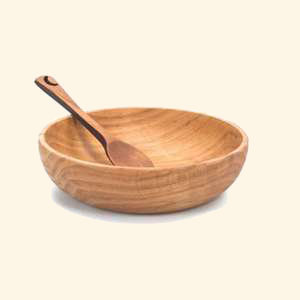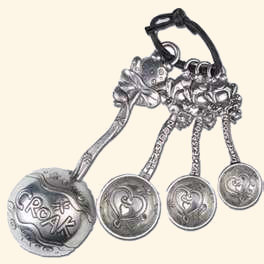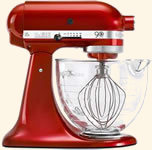Cooking Conversions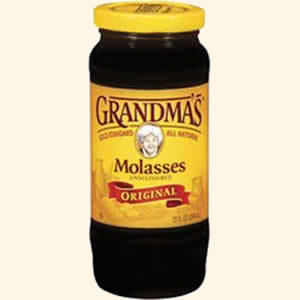# US Fluid Ounces to Imperial Fluid Ounces (US fl oz to UK fl oz)

As the US fluid ounce and the Imperial fluid ounce are not the same you will need to convert between the two when you have a recipe that is not in your home units. The US fluid ounce is slightly larger than the Imperial fluid ounce (unlike pints where the Imperial pint is larger) and there are only 16 US fluid ounces to the US pint whereas there are 20 Imperial fluid ounces to the Imperial pint.

Follow the links for conversion between US pints and Imperial pints and US fluid ounces to millilitres.

## US Fluid Ounces to Imperial Fluid Ounces Converter

Type a value in either field to convert from US fluid ounces to Imperial fluid ounces (US fl oz to UK fl oz) or Imperial fluid ounces to US fluid ounces(UK fl oz to US fl oz) (results are accurate to 2 decimal places).

 US Fluid oz UK Fluid oz US fl oz = UK fl oz

## Common US Fluid Ounce to Imperial Fluid Ounce Conversion Table

Common US fluid ounce to Imperial fluid ounce conversions used in cooking and baking are given in the table below (results are rounded to the 2 decimal places).

 US Fluid Ounces ¼ ½ ¾ 1 1½ 2 3 4 6 8 12 16 Imperial Fluid Ounces 0.26 0.52 0.78 1.04 1.56 2.08 3.12 4.16 6.25 8.33 12.49 16.65

## US Fluid Ounce to Imperial Fluid Ounce Conversion Formula

The conversion formula for US fluid ounces to Imperial fluid ounces (US fl oz to UK fl oz) is 1 US fluid ounce = 1.0408423 UK fluid ounces.

## Common Imperial Fluid Ounce to US Fluid Ounce Conversion Table

Values commonly used in cooking for Imperial fluid ounce to US fluid ounce conversions are given in the table below (results are rounded to 2 decimal places).

 Imperial Fluid Ounces ¼ ½ ¾ 1 1½ 2 3 4 5 10 15 20 US Fluid Ounces 0.24 0.48 0.72 0.96 1.44 1.92 2.88 3.84 4.8 9.61 14.41 19.22

## Imperial Fluid Ounce to US Fluid Ounce Conversion Formula

The conversion formula for Imperial fluid ounces to US fluid ounces (UK fl oz to US fl oz) is 1 Imperial fluid ounce = 0.9607603 US fluid ounces.

Other liquid measure conversions for cooking